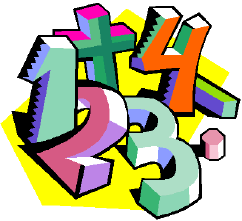# Sakib Knows The Number!

1,207.8K Views

Ragib: I got digits of a 2 digit number
Sakib: Is it an odd?
Ragib: Yes. Moreover, the sum of the digits of the number is a prime!
Sakib: The difference of the digits of the number is also a prime?
Ragib: Of course! Last of all, the multiply of the digits is equal to one of the digits!!
Sakib: Oh no! I know the number. It’s your roll number!
What is that number!The digits may be 1,2,3,4,5,6,7,8,9,0.
Since two digit number, 0 can only be put in the second digit position.
But that will make the number even. So 0 is not any of the digits!!
1* any digit=1 or the digit
2*any digit(not 1) = not 2 or not the digit
3*any digit(not 1) = not 3 or not the digit
4*any digit(not 1) = not 4 or not the digit
5*any digit(not 1) = not 5 or not the digit
6*any digit(not 1) = not 6 or not the digit
7*any digit(not 1) = not 7 or not the digit
8*any digit(not 1) = not 8 or not the digit
9*any digit(not 1) = not 9 or not the digit
So its clear one digit is 1.
So the number may be 11,21,31,41,51,61,71,81,91.
But the sum of the 2 digit is also a prime!
So 31,51,71,81,91 will be ignored!
Now the answer may be 11,21,41,61
But the difference of the digit also is a prime!!
So 11,21 will be ignored!!!!